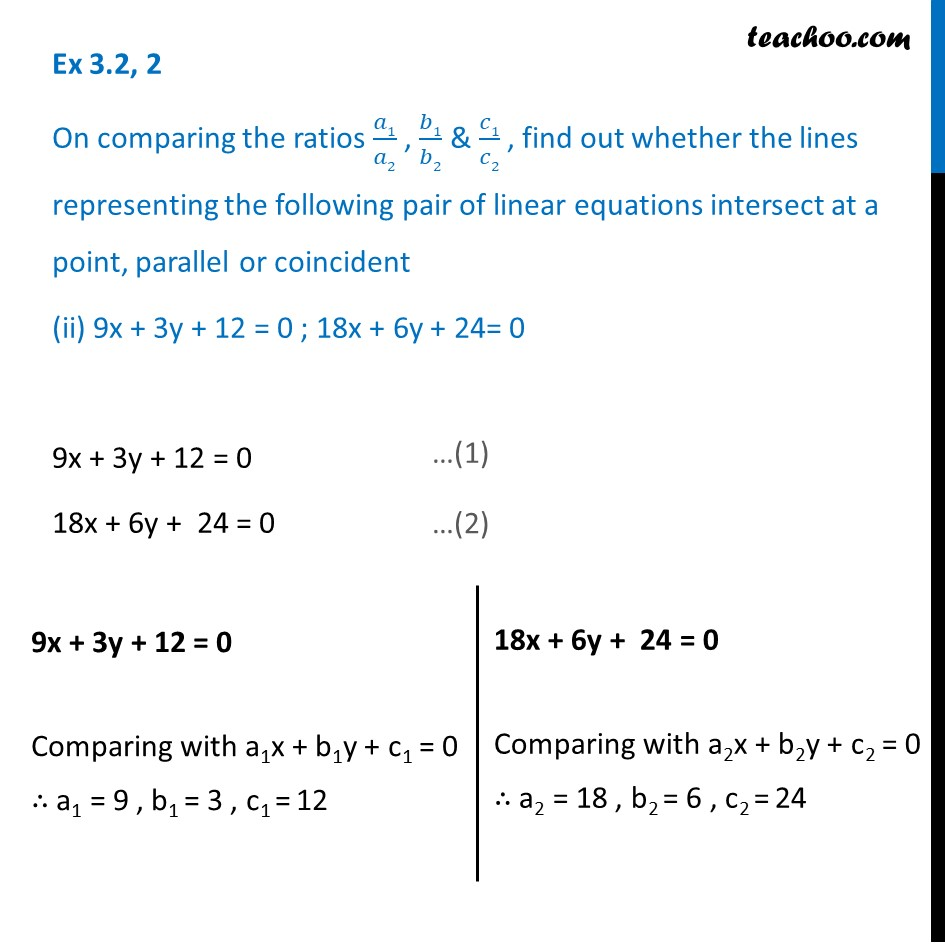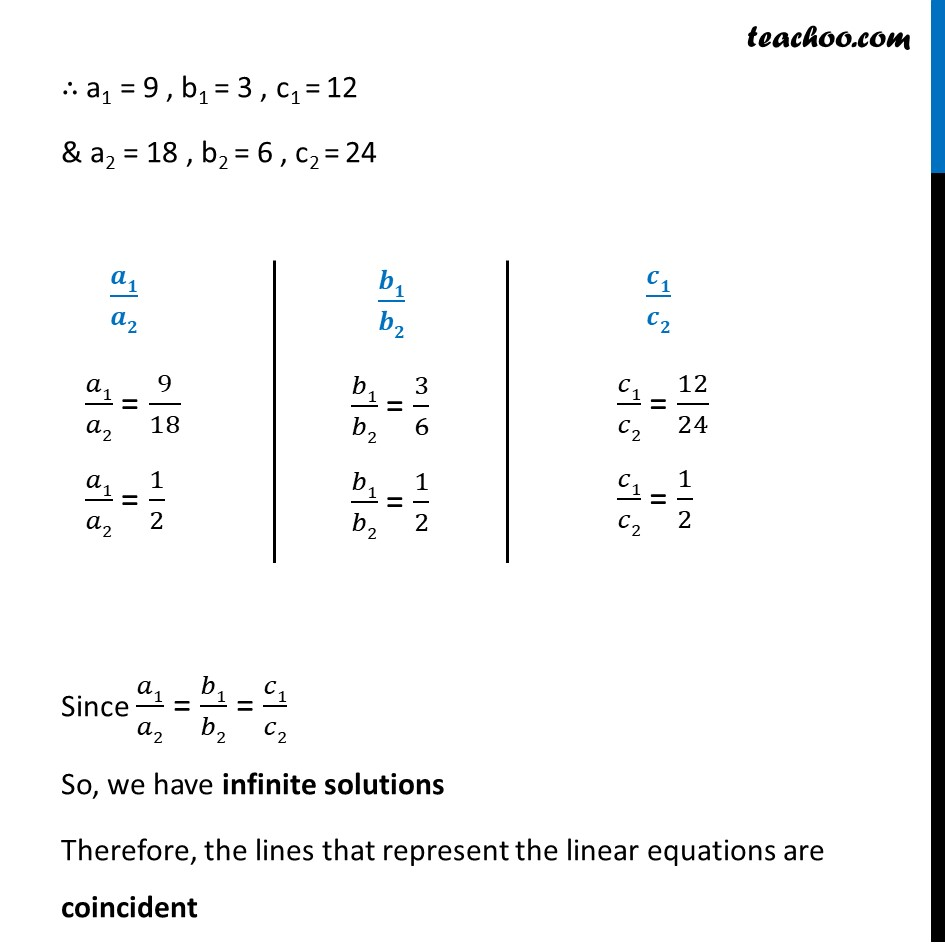1. Chapter 3 Class 10 Pair of Linear Equations in Two Variables (Term 1)
2. Serial order wise
3. Ex 3.2

Transcript

Ex 3.2, 2 On comparing the ratios 𝑎1/𝑎2 , 𝑏1/𝑏2 & 𝑐1/𝑐2 , find out whether the lines representing the following pair of linear equations intersect at a point, parallel or coincident (ii) 9x + 3y + 12 = 0 ; 18x + 6y + 24= 0 9x + 3y + 12 = 0 18x + 6y + 24 = 0 9x + 3y + 12 = 0 Comparing with a1x + b1y + c1 = 0 ∴ a1 = 9 , b1 = 3 , c1 = 12 18x + 6y + 24 = 0 Comparing with a2x + b2y + c2 = 0 ∴ a2 = 18 , b2 = 6 , c2 = 24 ∴ a1 = 9 , b1 = 3 , c1 = 12 & a2 = 18 , b2 = 6 , c2 = 24 𝒂𝟏/𝒂𝟐 𝑎1/𝑎2 = 9/18 𝑎1/𝑎2 = 1/2 𝒃𝟏/𝒃𝟐 𝑏1/𝑏2 = 3/6 𝑏1/𝑏2 = 1/2 𝒄𝟏/𝒄𝟐 𝑐1/𝑐2 = 12/24 𝑐1/𝑐2 = 1/2 Since 𝑎1/𝑎2 = 𝑏1/𝑏2 = 𝑐1/𝑐2 So, we have infinite solutions Therefore, the lines that represent the linear equations are coincident